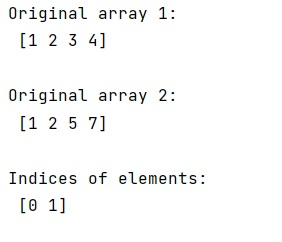# NumPy: For every element in one array, find the index in another array

Learn, how to find the index of an element of a numpy array by comparing the other numpy array?
Submitted by Pranit Sharma, on February 07, 2023

NumPy is an abbreviated form of Numerical Python. It is used for different types of scientific operations in python. Numpy is a vast library in python which is used for almost every kind of scientific or mathematical operation. It is itself an array which is a collection of various methods and functions for processing the arrays.

## Problem statement

Suppose that we are given two 1D arrays, x & y, one smaller than the other. We need to find the index of every element of y in x.

## Finding the index of an element of a numpy array by comparing the other numpy array

There is an easy approach to this problem. We will use numpy.in1d() method which is used to test whether each element of a 1-D array is also present in a second array.

It returns a boolean array the same length as ar1 that is True where an element of ar1 is in ar2 and False otherwise.

Let us understand with the help of an example,

## Python program to find the index of an element of a numpy array by comparing the other numpy array

```# Import numpy
import numpy as np

# Creating two numpy arrays
arr1 = np.array([1, 2, 3, 4])
arr2 = np.array([1, 2, 5, 7])

# Display original arrays
print("Original array 1:\n",arr1,"\n")
print("Original array 2:\n",arr2,"\n")

# Checking the indices of elements
# based on another array
res = np.where(np.in1d(arr1, arr2))

# Display the result
print("Indices of elements:\n",res,"\n")
```

## Output

The output of the above program is: